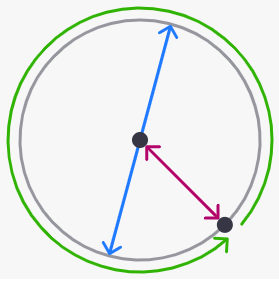安卓手机扫描二维码安装App

There are three important measurements related to circles that you need to know:

The radius is the distance from the center of a circle to its outer rim.

The diameter is the distance between two opposite points on a circle. It goes through its center, and its length is ( ？) the radius.

The circumference (or perimeter) is the distance around a circle.

A. half

B. the same as

C. twice苹果手机扫描二维码安装App